# ML Aggarwal Class 8 Solutions for ICSE Maths Model Question Paper 1

## ML Aggarwal Class 8 Solutions for ICSE Maths Model Question Paper 1

Choose the correct answer from the given four options (1-2):
Question 1.
Sum of rational number $$\frac { 5 }{ 7 }$$ and its additiveinverse is
(a) 1
(b) 0
(c) -1
(d) none of these
Solution: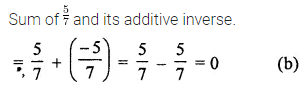Question 2.
Product of two rational numbers is 1. If one of them is $$\frac { 4 }{ 5 }$$, then other is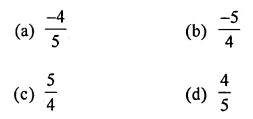Solution:Question 3.
Find the value of x for which $$\left(\frac{4}{9}\right)^{x} \times\left(\frac{3}{2}\right)^{-1}$$ = $$\frac{8}{27}$$.
Solution:Question 4.
Express the following numbers in standard form:
(i) 0.0000000000578
(ii) 345700000000000
Solution: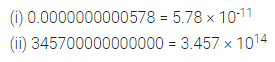Question 5.
Insert ten rational numbers between $$\frac{-4}{5}$$ and $$\frac{2}{3}$$.
Solution:Question 6.
Find the cube root of 50653.
Solution: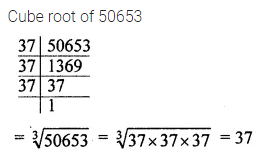Question 7.
Find the smallest number by which 3645 should be divided so that quotient is a perfect cube.
Solution:Question 8.
If p = $$\frac{-3}{5}$$, q = $$\frac{1}{2}$$, r= $$\frac{-7}{9}$$,then verify p × (q + r) = p × q + p × r.
Solution: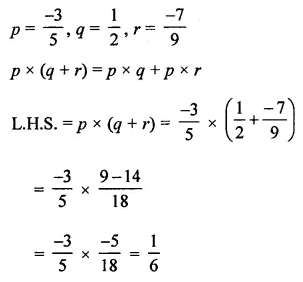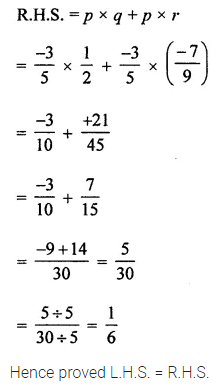Question 9.
Find the square root of 7056 by prime factorisation method.
Solution: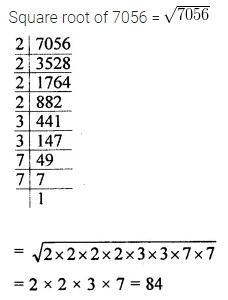Question 10.
Find the least number which must be added to 59000 to make it a perfect square.
Solution: